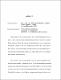## Analysis of models of superfluidityThis thesis deals with the rigorous analysis of two models of superfluidity. One of them is a macro-scale description of the interacting dynamics of a mixture of superfluid Helium and normal Helium. The equations used are modifications of the incompressible Navier-Stokes equations in 2D, with a nonlinear \textit{mutual friction} that couples the two fluids. We show global well-posedness of strong solutions (with high-regularity data) to this model, by proving a Beale-Kato-Majda-type condition. This work has been published in the Journal of Nonlinear Science. \\ Next, we study a micro-scale model (the Pitaevskii’’ model) of superfluid-normal fluid interactions, derived by Lev Pitaevskii in 1959. This involves the nonlinear Schr\"odinger equation and incompressible inhomogeneous Navier-Stokes equations. Mass and momentum exchange between the two fluids is mediated through a nonlinear and bidirectional coupling. We establish the existence of local solutions (strong in wavefunction and velocity, weak in density) that satisfy an energy equality. The analysis of this model has been published in the Journal of Mathematical Fluid Mechanics. \\ Finally, we prove a weak-strong type uniqueness theorem for the solutions of the Pitaevskii model. We begin by arguing that the standard weak-strong uniqueness argument does not seem to work in the case of weak solutions whose regularity is governed purely by the energy balance equation, even if the strong solution is as smooth as one wishes. Thus, we are forced to consider slightly less weak solutions obtained from a higher-order energy bound. Owing to their better regularity, we can compare them to \textit{moderate} solutions $-$ which are rougher than conventional strong solutions used for this purpose $-$ and establish a \textit{weak-moderate uniqueness} theorem. Relative to the solutions actually constructed in the earlier part of this thesis, only some of the regularity properties are used, allowing room for improved existence theorems in the future, while maintaining compatible uniqueness results. The uniqueness results have been accepted for publication in Nonlinearity.